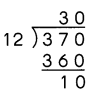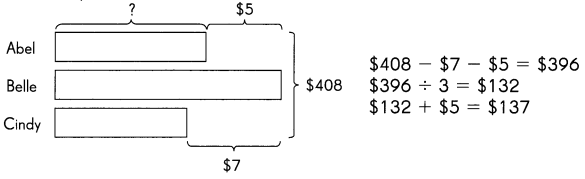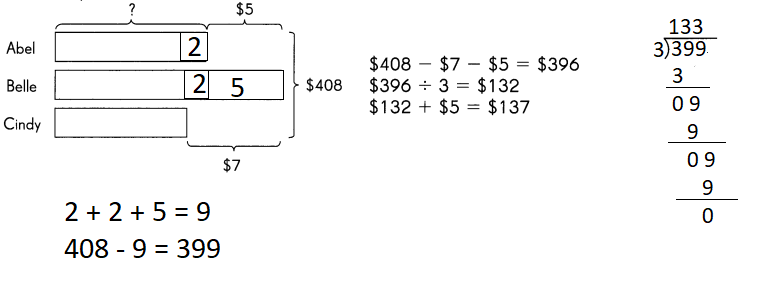# Math in Focus Grade 5 Chapter 2 Answer Key Whole Number and Multiplication and Division

## Math in Focus Grade 5 Chapter 2 Answer Key Whole Number and Multiplication and Division

Math Journal

Question 1.
Kelly has a 370-page sketch book. She wants to allocate an equal number of pages for making sketches to each month of the year. She uses division to find the number of pages she can possibly allocate to each month, and the number of pages she will have left over.
She works out the division like this:Which part of the answer tells the number of pages that Kelly can possibly allocate to each month?
Which part tells the number of pages left over?
Quotient
Remainder
Explanation:
Quotient part of the answer tells the number of pages that Kelly can possibly allocate to each month
Remainder part tells the number of pages left over

Question 2.
Mark was asked to simplify the numeric expression 6 + 4 × 2. He worked out the steps like this:
6 + 4 × 2 = 10 × 2
= 20
Is he correct? Explain why.
No, he is not correct
Explanation:
the answer will be 8 · 6+2=8 · Order or operations. You must multiply or divide before adding or subtracting

Question 3.
Look at the following problem and the solution given by a student: Abel, Belle, and Cindy have $408 altogether. Belle has$7 more than Cindy and $5 more than Abel. How much does Abel have?What was the mistake made? What should the correct answer be? Answer:Abel has 135 Explanation: Abel has 135 408 – 9 = 399 399 divided by 3 = 133 133 + 2 = 135 Put On Your Thinking Cap! Challenging Practice Solve. Use any strategy. Question 1. A sticker costs 15¢, and a packet of 8 similar stickers costs$1. Clement buys 37 stickers. What is the least amount of money that Clement spends on the stickers?
Answer: $4 and 75¢ Explanation: if buy Individual sticker: 37 x 15 = 555 =$5 and 5¢
37 ÷ 8 = 4 and remainder is 5
so, Clement needs to buy 4 packets and 5 stickers.
4 packets  cost is 4 x 1 = $4 and 5 stickers cost is 5 x 15 = 75 So total cost is =$4 75¢
least amount of money that Clement spends on the stickers is $4 and 75¢ Question 2. 40 members of a parents’ organization are making candles to raise money. 1 member drops out and the rest have to make 3 more candles each to make up. Each member makes the same number of candles. How many candles do they make altogether? Answer: 4680 Explanation: When possible, assign a variable to the value the problem wants you to find. Here, Let x=the total number of candies Let y = number of candies each when there are 40 members Translate: “40 members of a parents organization are making candles” and “Each member makes the same number of candles” means x=40*y “1 member drops out and the rest have to make 3 more candles each” means x=(40-1)*(y+3) “to make up” means that the same number of candies are made, so set the expressions equal: 40y = (40-1)(y+3) 40y = 39(y+3) 40y = 39y + 117 y = 117 So, x=40y x=40*117 x = 4680 Checking (very important): Is 40*117 = 39*(120) ? 4680 = 4680 ? yes Solve. Use any Strategy. Question 3. Mr. Thomas puts up fence posts from one end of a field to the other, equal distances apart. There are 27 posts. The width of each post is 10 centimeters. The distance between two posts is 30 meters. Find the length of the fence. Answer: 782 meters and 70 centimeters Explanation: There are 27 posts and puts up fence posts from one end of a field to the other, equal distances apart So total distances are 27 -1 = 26 The distance between two posts is 30 meters then total distance between posts is 26 x 30 = 780 meters The width of each post is 10 centimeters and then total posts width is 27 x 10 =270 centimeters = 2 meters and 70 centimeters Question 4. Kirsten has 64 coins in her piggy bank. She has$9.25 in dimes and quarters. How many dimes and how many quarters does she have?
Answer: 45 dimes and 19 quarters
Explanation:
Let there be x dimes and 64-x quarters.
0.1x+0.25(64-x) = 9.25
0.1x+16-0.25x=9.25
-0.15x=-6.75
x= 45
64-x=64-45=19
so 45 dimes and 19 quarters

Problem Solving

Solve. Use any strategy.

Question 1.
Darcy, Jason, and Maria share $268. Jason has$20 more than Darcy and Maria has twice as much money as Jason. How much money do Darcy and Jason have altogether?
Answer: Darcy and Jason have altogether=$124 Explanation: Let Darcy has money=x Jason has$20 more than Darcy
Jason =20+x
Maria has twice as much money as Jason
Maria = 2(20+x)=40+2x
Darcy,Jason and Maria share $268 x+20+x+40+2x=268 4x+60=268 4x=208 x=52 So Darcy has money=$52
Jason has money=20+x=20+52=$72 Darcy and Jason have altogether=$(52+72)=\$124

Question 2.
Juan and Rachel have the same number of marbles. Rachel gives away 10 marbles and Juan gives away 22 marbles. Rachel then has 3 times as many marbles as Juan. How many marbles did each of them have at first?
Explanation:
Let X = the number of marbles that Juan or Rachel started with.
Rachel gives away 10 marbles–> X – 10 and Juan gives away 22–> X – 22
Than Rachel has 3 times as many marbles as Juan–> X-10 = 3(X-22)
X – 10 = 3X – 66
-56 =- 2X
X = 28

Solve. Use any strategy.

Question 3.
Gerry had a total of 30 pens and pencils. He decided to trade with his friends all his pens for pencils. If he traded every pen for 2 pencils, he would have 48 pencils in all. How many pens and how many pencils did he have before the trade?
Explanation:
Let p = pens
Pencils = 30-p
Total pencils after the exchange will be: 48 = 30-p + 2p
48 = 30 + p
18 = p (pens)
30-18 = 12 pencils
To check: 18×2 + 12 = 36+12 = 48

Math Journal

184 × 97
200 × 100 = 20000 So 17848 is nearest number. It is reasonable.
Explanation:
184 × 97  = 17848

Math Journal

Jodi estimated these products.
a. 2,892 × 21 rounds to 3,000 × 20 = 60,000
b. 2,743 × 18 rounds to 3,000 × 20 = 60,000
She then worked out the actual answers. Even though the estimated answers were the same, Jodi found that the actual answers were very different from each other.
2,892 × 21 =60732
2,743 × 18  =  49374

Question 1.
In which case is the estimate closer to the actual answer? Explain why.